• 用VFP建立一元线性回归预测模型的论文，里面有详细程序，可以直接使用
• 一元线性回归预测法是分析一个因变量与一个自变量之间的线性关系的预测方法。 常用统计指标：平均数、增减量、平均...一元线性回归预测模型的建立 1、选取一元线性回归模型的变量 ； 2、绘制计算表和拟合散点图 ；
一元线性回归预测法是分析一个因变量与一个自变量之间的线性关系的预测方法。 常用统计指标：平均数、增减量、平均增减量

一元线性回归预测基本思想
确定直线的方法是最小二乘法 最小二乘法的基本思想：最有代表性的直线应该是直线到各点的距离最近。然后用这条直线进行预测。

一元线性回归预测模型的建立

1、选取一元线性回归模型的变量 ；
2、绘制计算表和拟合散点图 ；
3、计算变量间的回归系数及其相关的显著性 ；
4、回归分析结果的应用 。

模型的检验

1、经济意义检验：就是根据模型中各个参数的经济含义，分析各参数的值是否与分析对象的经济含义相符。
2、回归标准差检验
3、拟合优度检验
4、回归系数的显著性检验

利用回归预测模型进行预测

可以分为：点预测和置信区间预测法
1、点预测法：将自变量取值带入回归预测模型求出因变量的预测值。
2、置信区间预测法：估计一个范围，并确定该范围出现的概率。置信区间的大小的影响的因素：a、因变量估计值；b、回归标准差；C、概率度t；

一元线性回归分析预测法模型分析

一元线性回归分析预测法，是根据自变量x和因变量Y的相关关系，建立x与Y的线性回归方程进行预测的方法。由于市场现象一般是受多种因素的影响，而并不是仅仅受一个因素的影响。所以应用一元线性回归分析预测法，必须对影响市场现象的多种因素做全面分析。只有当诸多的影响因素中，确实存在一个对因变量影响作用明显高于其他因素的变量，才能将它作为自变量，应用一元相关回归分析市场预测法进行预测。
一元线性回归分析法的预测模型为：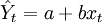$\hat{Y}_t=a+bx_t$　　　　（1）
式中，xt代表t期自变量的值；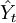$\hat{Y}_t$代表t期因变量的值；
a、b代表一元线性回归方程的参数。
a、b参数由下列公式求得（∑代表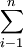$\sum^{n}_{i-1}$）：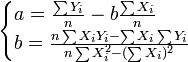$\begin{cases}a=\frac{\sum Y_i}{n}-b\frac{\sum X_i}{n}\\b=\frac{n\sum X_{i}Y_{i}-\sum X_i\sum Y_i}{n\sum X^2_i-(\sum X_i)^2} \end{cases}$
为简便计算，我们作以下定义：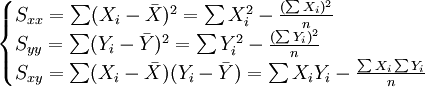$\begin{cases}S_{xx}=\sum(X_i-\bar{X})^2=\sum X^2_i-\frac{(\sum X_i)^2}{n}\\S_{yy}=\sum(Y_i-\bar{Y})^2=\sum Y^2_i-\frac{(\sum Y_i)^2}{n}\\S_{xy}=\sum(X_i-\bar{X})(Y_i-\bar{Y})=\sum X_i Y_i-\frac{\sum X_i\sum Y_i}{n}\end{cases}$　　　　(2)
式中：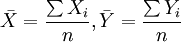$\bar{X}=\frac{\sum X_i}{n},\bar{Y}=\frac{\sum Y_i}{n}$
这样定义a、b后，参数由下列公式求得：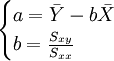$\begin{cases}a=\bar{Y}-b\bar{X}\\b=\frac{S_{xy}}{S_{xx}}\end{cases}$　　　　(3)
将a、b代入一元线性回归方程Yt = a + bxt，就可以建立预测模型，那么，只要给定xt值，即可求出预测值$\hat{Y}_t$。
在回归分析预测法中，需要对Ｘ、Ｙ之间相关程度作出判断，这就要计算相关系数r，其公式如下：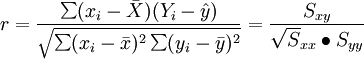$r=\frac{\sum(x_i-\bar{X})(Y_i-\hat{y})}{\sqrt{\sum(x_i-\bar{x})^2\sum(y_i-\bar{y})^2}}=\frac{S_{xy}}{\sqrt S_{xx}\bullet{S_{yy}}}$
相关系数r的特征有：
①相关系数取值范围为：-1≤r≤1 。
②r与b符合相同。当r>0，称正线性相关，Xi上升，Yi呈线性增加。当r<0，称负线性相关，Xi上升，Yi呈线性减少。
③|r|=0，X与Y无线性相关关系；|r|=1，完全确定的线性相关关系；0<|r|<1，X与Y存在一定的线性相关关系；|r|>0.7，为高度线性相关；0.3<|r|≤0.7，为中度线性相关；|r|≤0.3，为低度线性相关。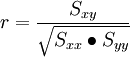$r=\frac{S_{xy}}{\sqrt{S_{xx}\bullet S_{yy}}}$　　　　(4)


展开全文• 一元线性回归分析模型在家庭消费支出预测中的应用，高玉，周树民，介绍一元线性回归分析的基本概念和方法原理，并以2001年到2010年国民的城镇居民家庭人均支配收入（简称
• 基于修正的一元线性回归模型的企业利润预测研究，张琛，那宝贵，本文针对一元线性回归模型进行研究，用马尔可夫链状模型一元线性回归模型进行修正和改进，使得修正后的一元线性回归模型更加的
• 本文主要介绍线性回归模型，该模型主要应用于监督学习中目标变量是连续数值型的场景。...最简单的线性回归模型就是一元线性回归模型，其模型的形式就是： y=ax+b(1) y=ax+b \tag{1} y=ax+b(1) 由式...
本文主要介绍线性回归模型，该模型主要应用于监督学习中目标变量是连续数值型的场景。
一元线性回归模型
线性回归模型是数据科学领域最简单的模型，很多复杂的模型 （如多项式回归、逻辑回归、SVM） 都是建立在线性回归模型的基础上的，或者是从其中能找到线性回归模型的影子。最简单的线性回归模型就是一元线性回归模型，其模型的形式就是：

y

=

a

x

+

b

(1)

y=ax+b \tag{1}

由式(1)可知，线性回归是针对连续数值型变量的模型，且样本只有一个特征，即只有一个自变量。所谓线性，是指方程是线性的，也就是在寻找一条直线来拟合数据；所谓回归，是指用方程来模拟变量之间是如何关联的。线性回归就是要找一条直线，并且让这条直线尽可能地拟合训练样本中的数据点。
虽然我们可以找到一条拟合训练集的直线，但是同一训练样本可以拟合出很多条直线，我们如何判断那条直线是最适合的？这就引出了如何评价模型结果的好坏，又通过什么样的方式来判断？接下来我们通过损失函数来了解线性回归的评价标准。
损失函数
为了衡量模型的效果，很自然的就会想到衡量预测值与真实值之间的差别。假设预测值为f(x)，真实值为y，样本的数量为n，那么就有：

∑

i

=

1

n

(

f

(

x

i

)

−

y

i

)

2

(2)

\sum_{i=1}^n(f(x_i)-y_i)^2 \tag{2}

也就是每一个样本的预测值与真实值差的平方和，取平方是为了消除负数的影响。
式(2)就是均方误差(MSE, Mean Squared Error)，它对应了常用的欧氏距离（也就是计算两点之间距离）。均方误差是机器学习中回归问题经常使用的评价方式，在机器学习中称之为损失函数。可以看到一个公式在不同的地方会有不同的名字，很容易搞混，但我们只要理解其中的基本思想就会很清楚了。
所以，现在我们知道了损失函数是衡量回归模型误差的函数，也就是我们要的“直线”的评价标准。这个函数的值越小，说明直线越能拟合我们的数据。
得到损失函数后，又如何求得使损失函数最小的a和b呢，接下来我们通过最小二乘法来获得问题的解。
最小二乘法
回归方程里，求得特征对应的最佳回归系数的方法是最小化误差的平方和，也就是损失函数。
假设式(2)的值为S，将式(1)代入式(2)可得：

S

=

∑

i

=

1

n

(

f

(

x

i

)

−

a

x

i

−

b

)

2

(3)

S =\sum_{i=1}^n(f(x_i)-ax_i-b)^2 \tag{3}

在xi和yi已知的前提下，式(3)是a和b的二次函数。由微积分的知识可知，要求得a和b的最小值分别对a和b求偏导，当两者的偏导数为0时取得最小值。如下所示：

∂

S

∂

a

=

2

∑

i

=

1

n

(

y

i

−

a

x

i

−

b

)

x

i

=

0

(4)

\frac {\partial S } {\partial a }=2\sum_{i=1}^n(y_i - ax_i-b)x_i = 0 \tag{4}

∂

S

∂

b

=

2

∑

i

=

1

n

(

y

i

−

a

x

i

−

b

)

=

0

(5)

\frac{\partial S}{\partial b} = 2\sum_{i=1}^n(y_i-ax_i-b)=0 \tag{5}

将xi和yi代入(4)和(5)式中就可以得到a和b的最小值。
总结
本文简单介绍了一元线性回归模型及其损失函数，并通过最小二乘法求得线性方程的最小参数。
参考资料
模型之母：简单线性回归&最小二乘法
用人话讲明白线性回归LinearRegression
机器学习眼中的线性回归
展开全文损失函数 机器学习 最小二乘法
• 即统计回归模型的具体形式和模型参数的估计值 对这些定量关系式的可信度进行统计检验 判别和选择诸因素中重要的影响因素 对经济活动进行分析和预测 3、回归与因果关系 虽然回归分析研究一个变量对另一（些）变量...
• 一元线性回归模型很简单y1=ax+b+ε，y1为实际值，ε为正态的误差。y2=ax+b，y2为预测值。ε=y1-y2。def model(a,b,x):# x is vector，a and b are the common number.return a*x+b这里将整组数据的预测结果方差作为...
一元线性回归模型很简单
y1=ax+b+ε，y1为实际值，ε为正态的误差。
y2=ax+b，y2为预测值。
ε=y1-y2。def model(a,b,x):
# x is vector，a and b are the common number.
return a*x+b
这里将整组数据的预测结果方差作为损失函数。
J(a,b)=sum((y1-y2)^2)/ndef cost(a,b,x,y):
# x is argu, y is actual result.
n=len(x)
return np.square(y-a*x-b).sum()/n
优化函数则进行使损失函数，即方差最小的方向进行搜索
a=a-theta*(∂J/∂a)
b=b-theta*(∂J/∂b)
这里的解释就是，对影响因素a或b求损失函数J的偏导，如果损失函数随着a或b增大而增大，我们就需要反方向搜索，使得损失函数变小。
对于偏导的求取则看的别人的推导公式
theta为搜索步长，影响速度和精度(我猜的，实际没有验证)def optimize(a,b,x,y):
theta = 1e-1 # settle the step as 0.1
n=len(x)
y_hat = model(a,b,x)
# compute forecast values
da = ((y_hat-y)*x).sum()/n
db = (y_hat-y).sum()/n
a = a - theta*da
b = b - theta*db
return a, b
使用sklearn库，可以使用现成的线性回归模型import numpy as np
from sklearn.linear_model import LinearRegression
import matplotlib.pyplot as plt
x = [1,2,4,5,6,6,7,9]
x = np.reshape(x,newshape=(8,1))
y = [10,9,9,9,7,5,3,1]
y = np.reshape(y,newshape=(8,1))
# create an instance of LinearRegression model
lr = LinearRegression()
# train model
lr.fit(x,y)
lr.score(x,y)
# compute y_hat
y_hat = lr.predict(x)
# show the result
plt.scatter(x,y)
plt.plot(x, y_hat)
plt.show()
展开全文• ## 线性回归预测模型

千次阅读 2019-08-09 15:36:30
一元线性回归模型： ε：模型误差项，平衡等号两边值 import seaborn as sns income = pd.read_csv(r'Salary_Date.csv') sns.lmplot(x='YearExperience',y='Salary', data=income,ci=None) plt.show() 线性...
一元线性回归模型：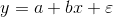ε：模型误差项，平衡等号两边值
import seaborn as sns
sns.lmplot(x='YearExperience',y='Salary',
data=income,ci=None)
plt.show()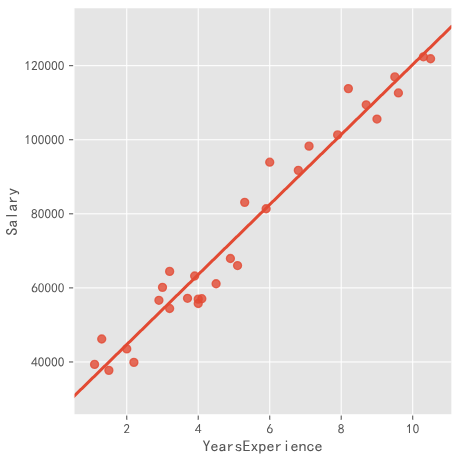线性拟合求解： 误差项最小，转换为误差平方项最小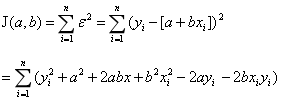最小时，偏导数为0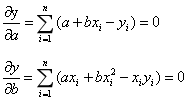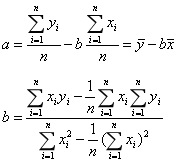①使用基本语法求解
n = income.shape
sum_x = income.YearsExperience.sum()
sum_y = income.Salary.sum()
sum_x2 = income.YearsExperience.pow(2).sum()
xy = income.YearsExperience * income.Salary
sum_xy = xy.sum()
b = (sum_xy - sum_x * sum_y / n) / (sum_x2 - sum_x ** 2 / n)
a = sum_y.mean() - b * sum_x.mean()

②使用statsmodels中的ols函数 ols(formula,data,subset=None,drop_cols) formula：‘y~x’ subset：bool类型，子集建模
import statsmodels.api as sm
fit = sm.formula.ols('income.Salary ~ income.YearsExperience',data=income).fit()
fit.params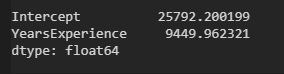多元线性回归
构建多元线性回归的数据集包含n个观测，p+1个变量（p个自变量，1个因变量）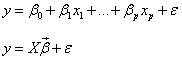β：p1的一维向量，偏回归系数 ε：n1的一维向量，误差项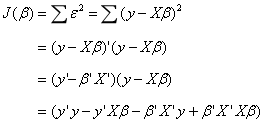常量，转置为本身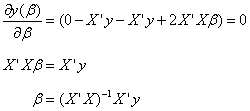Prodict_Profit.xlsx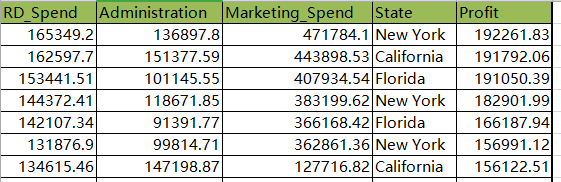State为字符型离散变量
from sklearn import model_selection
import statsmodels.api as sm
train,test = model_selection.train_test_split(profit,test_size=0.2,random_state=1234)
model = sm.formula.ols('Profit ~ RD_Spend + Administration + Marketing_Spend + C(State)',data=train).fit()
print(model.params)
test_X = test.drop(labels='Profit',axis=1)
pred = model.predict(exog=test.X)
print({'prediction':pred,'real':test.Porfit})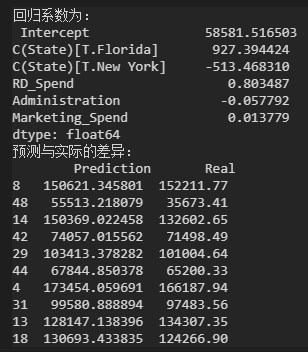State为离散变量，建模时为哑变量，嵌套在C()，表示为category变量。 State：Cal，Flo，NY。模型中默认将Cal作为对照组。
将NY作为对照组
dummies = pd.get_dummies(profit.State)
profit_new = pd.concat([profit,dummies],axis=1)
profit_new.drop(labels=['State','New York'],axis=1,inplace=True)
train,test = model_selection.train_test_split(profit_new,test_size=0.2,random_state=1234)
model2 = sm.formula.ols('Profit ~  RD_Spend + Administration + Marketing_Spend + Flo + Cal',data=train).fit()
print(model2.params)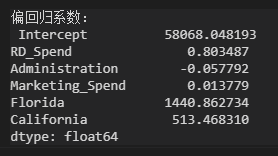从state中演变出来的哑变量在回归系数中只保留了Flo和Cal，而NY作为参照组。 以RD_Spend和Fla为例：在其他变量不变的情况下，研发成本每增加1元，利润增加0.8元；在其他不变的情况下，以NY为基准，在Flo销售，增加1440元。
回归模型的假设检验
模型的显著性检验是构成因变量的线性组合是否有效，即模型中是否存在一个自变量能够真正影响到因变量的波动，整体的效应。 回归系数的显著性检验是说明单个自变量在模型中是否有效。
模型显著性和回归系数显著性分别使用统计学中的F检验，t检验。
F检验
①提出问题原假设和备选假设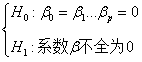通过数据推翻H0，接受H1 ②构造统计量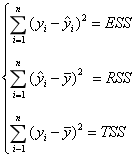ESS：误差平方和，随时间变动 RSS：回归离差平方和，随时间变动 TSS：总离差平方和，不变 TSS=ESS+RSS 线性回归模型的参数求解，使ESS最小，则RSS最大。
构造F统计量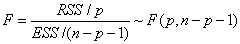p：RSS自由度 n-p-1：ESS自由度
③
import numpy as np
ybar = train.Profit.mean()
p = model2.df_model
n = train.shape
RSS = np.sum((model2.fittedvalues - ybar) **2)
ESS = np.sum(model2.resid **2)
F = (RSS / p) / (ESS / (n-p-1))
print(F)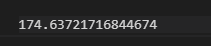#验证手动计算值是否正确
model2.fvalue

④对比F统计量的值和理论F分布的值
#调用计算理论分布值
from scipy.stats import f
F_throry = f.ppf(q=0.95,dfn=p,dfd=n-p-1)
print(F_theory)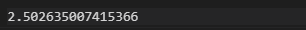F统计量值远远大于F分布的理论值，所以拒绝原假设，认为多元线性回归模型是显著的，所以回归模型的偏回归系数不全为0。
回归系数的显著性检验，t检验 ①提出假设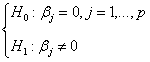原假设：第j变量的偏回归系数为0
②构造统计量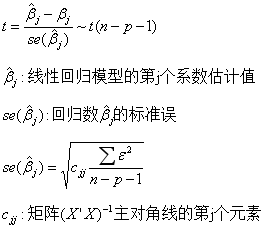③计算统计量
model2.summary()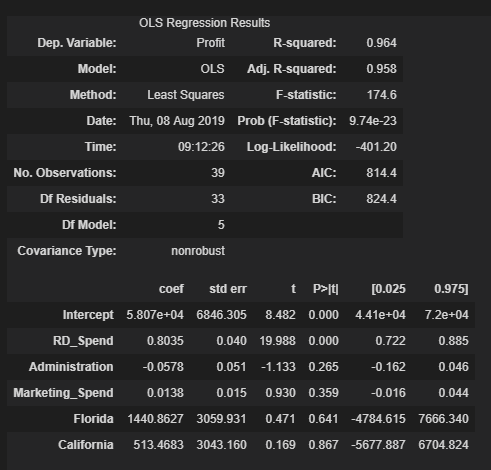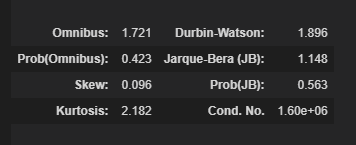第一部分： 模型的判决系数R-squared：自变量对因变量的解释程度 模型的F-statistic：检验模型的显著性 模型的信息准则AIC，BIC：对比模型的拟合效果好坏 第二部分： 回归系数的估计值Coef，t统计量值，回归系数的置信区间 第三部分： 模型误差项ε的有关信息，检验误差项独立性的杜宾-瓦特森统计量Durbin-Watson，衡量误差项是否服从正态分布的JB统计量，误差项偏度Skew，峰度Kurtosis
④第二部分含有每个偏回归系数的t统计量值，由估计值Coef和标准误Std err的商所得，每个t统计量都对应了概率值p，用来判别统计量是否显著的直接方法，通常概率p小于0.05时表示拒绝原假设，未通过表示变量不是重要因素。
检验通过后，开始对模型进行诊断
回归模型的诊断
模型的假设对残差项要求服从正态分布，实质要求因变量服从正态分布。 正态性检验运用两种方法： 定性的图形法 定量的非参数法
①直方图法
import scipy.stats as stats
plt.rcParams['font.sans-serif'] = ['SimHei']
plt.rcParams['axes.unicode-minus'] = False
plt.style.use('ggplot')
sns.distplot(a=profit_new.Profit,bins=10,fit=stats.norm,norm_hist=True,
hist_kws=['color':'steelblue','edgecolor':'blue'],
kde_kws=['color':'orange','linestyle':'-','label':True],
fit_kws=['color':red,'linestyle':'--'])
plt.legend()
plt.show()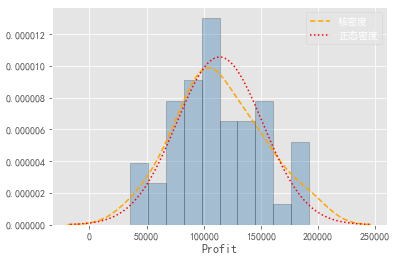绘制变量Profit的直方图，核密度曲线，理论正态分布，如果两条曲线近似或者吻合，说明服从正态分布。
②PP图，QQ图
#残差的正态检验
import ststamodels.api as sm
pp_qq_plot = sm.ProbPlot(profit_new.Profit)
#PP图
pp_qq_plot.ppplot(line=45)
plt.title('PP图')
pp_qq_plot.qqplot(line='q')
plt.title('QQ图')
plt.show()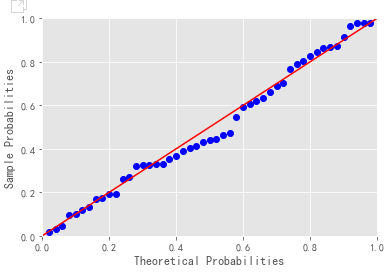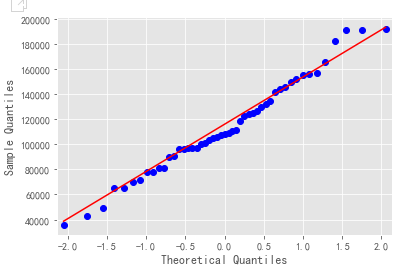PP图：对比正态分布的累计概率值和实际分布的累计概率值 QQ图：对比正态分布的分位数和世纪分布的分位数 判断变量是否服从正态分布的标准：散点都比较均匀的落在直线上。
③Shapiro K-S 假设变量服从正态分布，若数据小于5000，使用shapiro检验
import scipy.stats as stats
stats.shapiro(profit_new.Profit)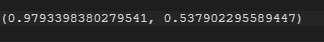返回元组，第一个元素是shapiro检验的统计量值，第二个元素是对应的概率p，由于p值大于置信水平0.05，故接受利润分布服从正态分布
#生成正态分布和均匀分布
rnorm = np.random.normal(loc=5,scale=2,size=1000)
runif = np.random.unifrom(low=1,high=100,size=1000)
#正态性检验
KS_test1 = stats.kstest(rvs=rnorm,args=(rnorm.mean(),rnorm.std()),cdf='norm')
KS_test2 = stats.kstest(rvs=runif,args=(runif.mean(),runif.std()),cdf='norm')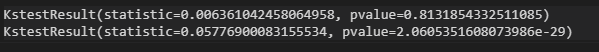正态随机分布随机数的检验p值大于置信水平0.05，接受原假设 均匀分布p值小于0.05，拒绝原假设 使用kstest函数对变量进行正态性检验，必须指定args参数，传递被检验变量的均值和标准差 变量检验成果不满足正态分布，使用数学转换。
展开全文• 一元线性回归是描述两个变量之间的相关关系最简单的模型。 根据两个变量之间的相关关系，拟合出一条直线来预测因变量变化的轨迹。 下面通过自己模拟的一些数据都进行模型预测 预测值的轨迹 import pandas as pd ...
• 我们可以通过回归方程（回归预测模型）来预测因变量的变化。回归分析的分类：1） 按自变量的个数，可以分为一元回归，多元回归2）按变量相关性的形状（回归线）是否为直线型，可分为线性回归，非线性回归。下面，...
• -*-coding:utf-8-*-import numpy as npimport matplotlib.pyplot as plt2、定义线性回归模型def predict(x, a, b):y_predict = a * x + breturn y_predict一元线性回归模型如下：公式1—一元线性回归模型损失函数...
• 一元线性回归分析预测模型，根据历史数据预测下期数据值
• x_d)$，其中$x_i$为$\boldsymbol{x}$在第$i$个属性上的取值，线性模型（linear model）试图学得一个通过属性的线性组合来进行预测的函数，即：$\$f(\boldsymbol{x}) = w_1x_1 + w_2x_2 + ... +...
• 之前的博文讲的是理论。现在需要用到成熟的库，来应用。 Science 科学的工具包 kit # sklearn 命名惯例： ...# LinearRegression 线性回归的类，我们可以使用该类实现线性回归。 # 底层就是使用...机器学习 sklearn
• 线性回归模型属于经典的统计学模型，该模型的应用...一元线性回归模型：y=a+bx+ε import pandas as pd import matplotlib.pyplot as plt import seaborn as sns f = open(r'C:\Users\active\Music\Desktop\Pyt...python 机器学习 人工智能 tensorflow
• 简要：实际上计量模型估计和实现最好用的软件还是stata，这个软件无论在国内还是国外，经济学家们都是爱不释手。今天我们要介绍的是python中的一个较为强大的统计分析包(Statsmodels)，不过需要强调的是python因为...
• 一元线性回归模型在电力系统负荷预测中的应用与MATLAB实现.pdf
• 为此提出了基于泛函网络的非线性回归预测模型和相应的学习算法。并分别就一元非线性回归预测和多元非线性回归预测给出了相应的实例。计算机仿真结果表明，泛函网络预测模型拟合度和预测精度都明显高于某些传统的方法...
• ,])14 lrModel.predict([,]) 小结： 一元n次方程，转换为多元线性方程： pf=sklearn.preprocessing.PolynomialFeatures(degree=2) 转换方法： x_2_fit=pf.fit_trasform(x) 预测： LinearRegression()....
• R语言中文社区专栏作者知乎ID：https://www.zhihu.com/people/herain-14前言数据挖掘的学习中，一元线性回归，通过现实生活中的企业销售和广告支出这两者之间的联系，进行线性回归模型的学习和形成商业二维变量分析...
• ## 第七章线性回归预测模型

千次阅读 多人点赞 2019-07-29 09:22:15
线性回归模型属于经典的统计学模型，该模型的应用场景是根据已知的变量（自变量）来预测某个连续的数值变量（因变量）。例如，餐厅根据每天的营业数据（包括菜谱价格、就餐人数、预定人数、特价菜折扣等）预测就餐...python
• 一元线性回归模型 参数估计（最小二乘法） 最小二乘法c++实现 导言 监督学习中，预测的变量是离散的，则称分类 otherwise 连续的变量称回归。 回归中只包含一个自变量和因变量，二者为线性关系，则为一元线性...
• 一元线性回归与线性神经网络模型关联性分析，杨达，王会进，一元线性回归和线性神经网络模型在实际应用中都能进行预测，两者都是线性模型。为了探讨两者之间的关联，从两模型的具体学习出发神经网络...